# Fundamentals of Corporate Finance Chapter 6 Bond Valuation

• Slides: 42Fundamentals of Corporate Finance Chapter 6 Bond Valuation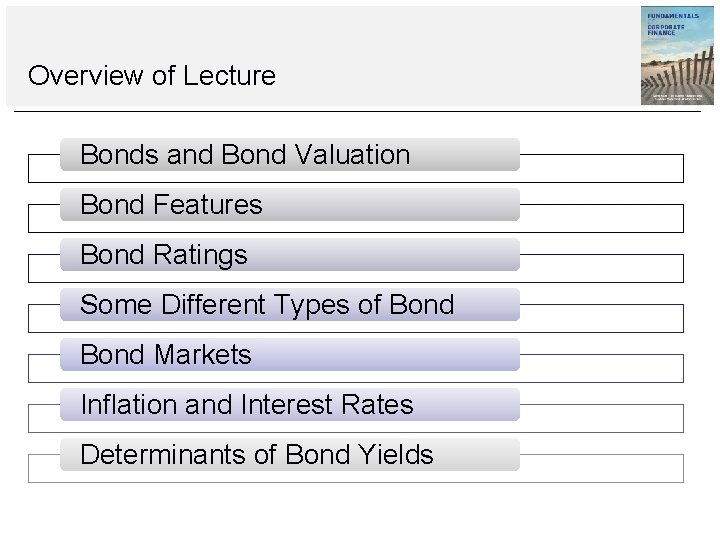Overview of Lecture Bonds and Bond Valuation Bond Features Bond Ratings Some Different Types of Bond Markets Inflation and Interest Rates Determinants of Bond YieldsCorporate Finance in the News Insert a current news story here to frame the material you will cover in the lecture.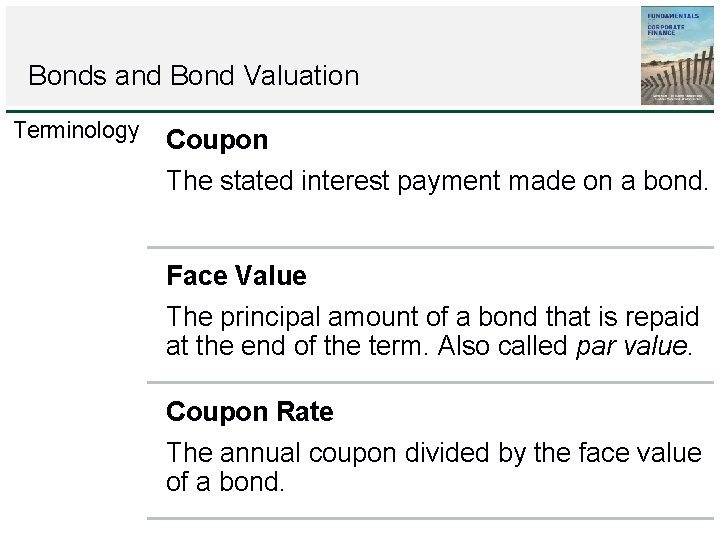Bonds and Bond Valuation Terminology Coupon The stated interest payment made on a bond. Face Value The principal amount of a bond that is repaid at the end of the term. Also called par value. Coupon Rate The annual coupon divided by the face value of a bond.Bonds and Bond Valuation Terminology Maturity The specified date on which the principal amount of a bond is paid. Yield to maturity (YTM) The rate required in the market on a bond.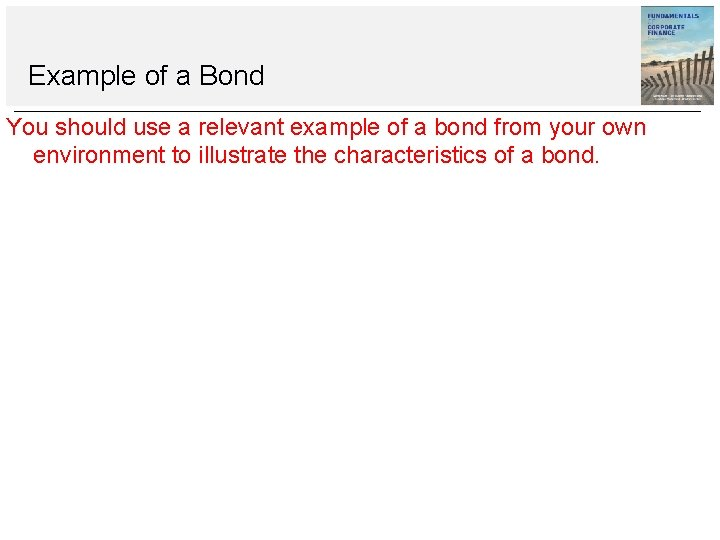Example of a Bond You should use a relevant example of a bond from your own environment to illustrate the characteristics of a bond.Bond Values and Yields Pixie plc plan to issue a bond with 10 years to maturity. The Pixie bond has an annual coupon of £ 80. Similar bonds have a yield to maturity of 8 per cent. What are the bond cash flows? What would this bond sell for?Bond Values and YieldsBond Valuation PV of Face Value Bond Value PV of AnnuityBond Values and Yields Pixie plc example PV of Face Value: Present value = £ 1, 000/1. 0810 = £ 1, 000/2. 1589 = £ 463. 19 PV of Annuity: Annuity present value = £ 80 (1 1/1. 0810)/. 08 = £ 80 (1 1/2. 1589)/. 08 = £ 80 6. 7101 = £ 536. 81 Bond Value: PV of Face Value + PV of Annuity = £ 463. 19 + £ 536. 81 = £ 1, 000Bond Values and Yields Bond Yield increases to 9% Bond Yield falls to 6% • What is the new bond value? • What is the new bond Value?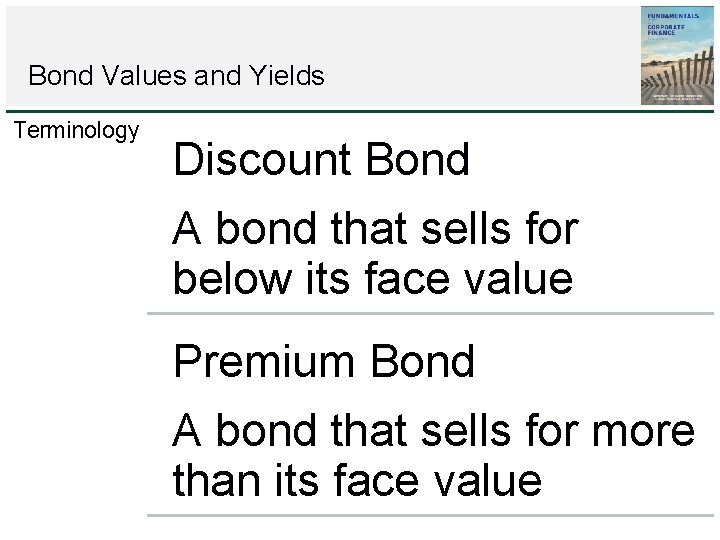Bond Values and Yields Terminology Discount Bond A bond that sells for below its face value Premium Bond A bond that sells for more than its face value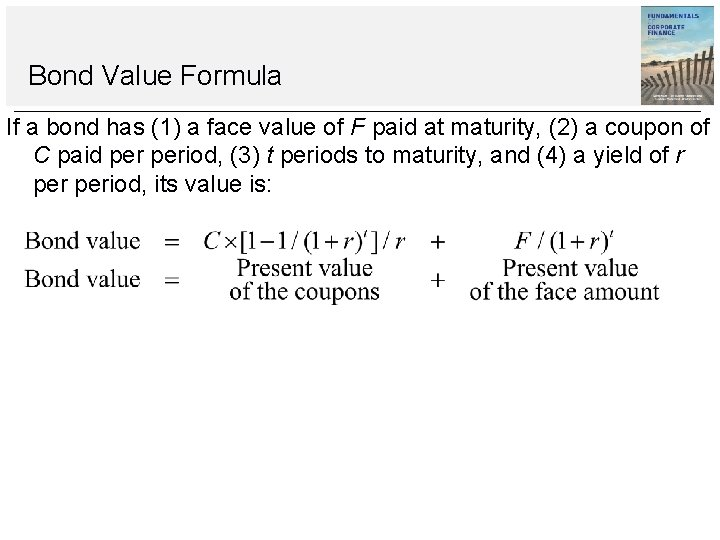Bond Value Formula If a bond has (1) a face value of F paid at maturity, (2) a coupon of C paid period, (3) t periods to maturity, and (4) a yield of r period, its value is:Example 6. 1 Semi-Annual Coupons Many bonds make coupon payments twice a year. So, if an ordinary bond has a coupon rate of 14 per cent and a face value of £ 100, 000, then the owner will get a total of £ 14, 000 per year, but this £ 14, 000 will come in two payments of £ 7, 000 each. Suppose we are examining such a bond. The yield to maturity is quoted at 16 per cent. Bond yields are presented in the same way as quoted rates, which is equal to the actual rate period multiplied by the number of periods. In this case, with a 16 per cent quoted yield and semiannual payments, the true yield is 8 per cent per six months. The bond matures in seven years. What is the bond’s price? What is the effective annual yield on this bond?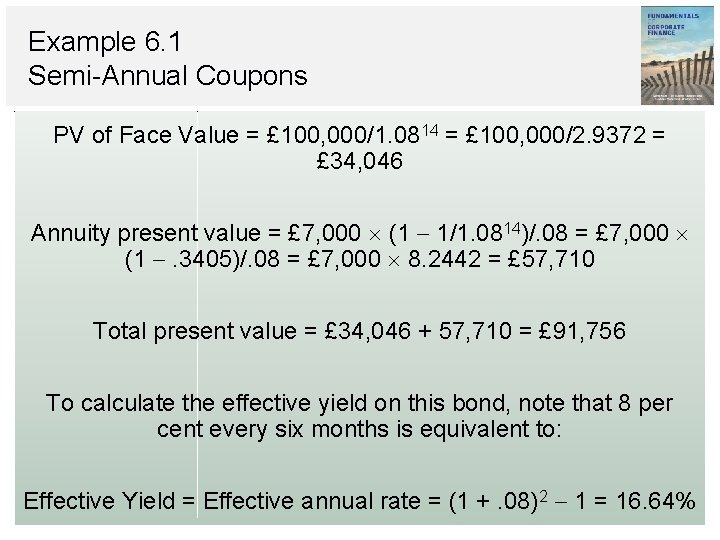Example 6. 1 Semi-Annual Coupons PV of Face Value = £ 100, 000/1. 0814 = £ 100, 000/2. 9372 = £ 34, 046 Annuity present value = £ 7, 000 (1 1/1. 0814)/. 08 = £ 7, 000 (1 . 3405)/. 08 = £ 7, 000 8. 2442 = £ 57, 710 Total present value = £ 34, 046 + 57, 710 = £ 91, 756 To calculate the effective yield on this bond, note that 8 per cent every six months is equivalent to: Effective Yield = Effective annual rate = (1 +. 08)2 1 = 16. 64%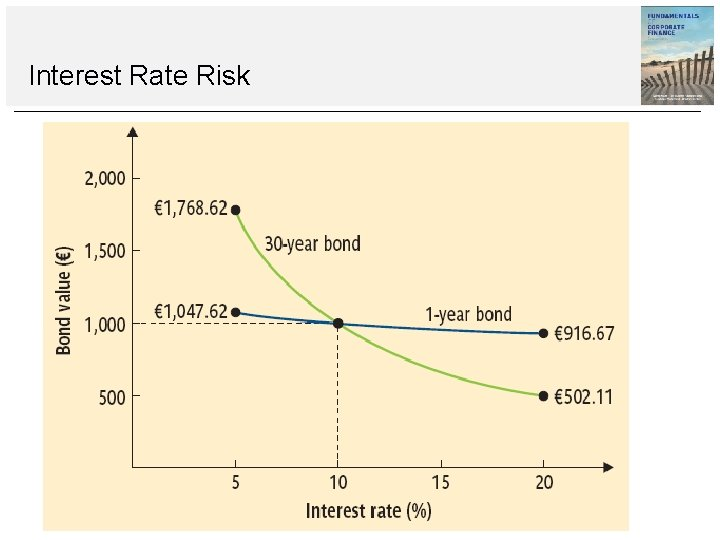Interest Rate RiskInterest Rate Risk All other things being equal, the longer the time to maturity, the greater the interest rate risk. All other things being equal, the lower the coupon rate, the greater the interest rate risk.Example 6. 2 Current Events A bond has a quoted price of £ 108, 042. It has a face value of £ 100, 000, a semiannual coupon of £ 3, 000, and a maturity of five years. What is its current yield? What is its yield to maturity? Which is bigger? Why?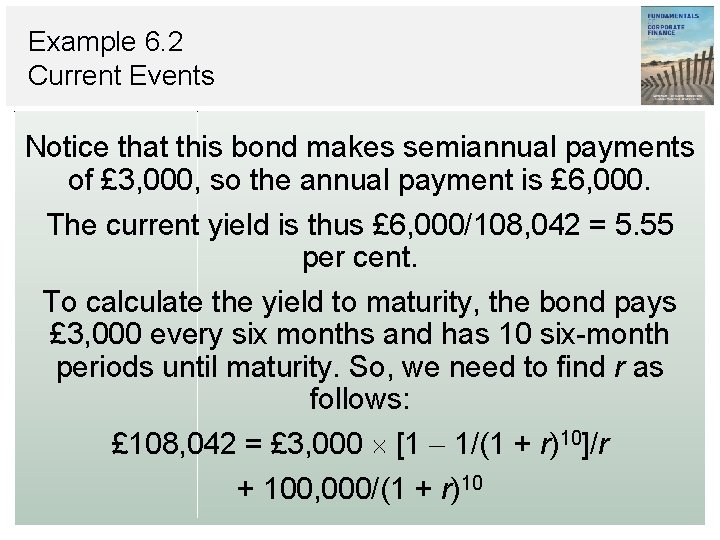Example 6. 2 Current Events Notice that this bond makes semiannual payments of £ 3, 000, so the annual payment is £ 6, 000. The current yield is thus £ 6, 000/108, 042 = 5. 55 per cent. To calculate the yield to maturity, the bond pays £ 3, 000 every six months and has 10 six-month periods until maturity. So, we need to find r as follows: £ 108, 042 = £ 3, 000 [1 1/(1 + r)10]/r + 100, 000/(1 + r)10Example 6. 2 Current Events After some trial and error, we find that r is equal to 2. 1 per cent. But, the tricky part is that this 2. 1 per cent is the yield per six months. We have to double it to get the yield to maturity, so the yield to maturity is 4. 2 per cent, which is less than the current yield. The reason is that the current yield ignores the built-in loss of the premium between now and maturity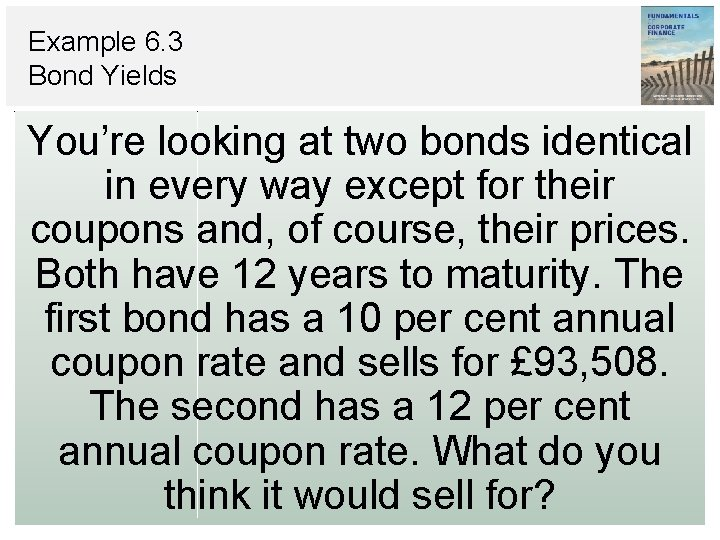Example 6. 3 Bond Yields You’re looking at two bonds identical in every way except for their coupons and, of course, their prices. Both have 12 years to maturity. The first bond has a 10 per cent annual coupon rate and sells for £ 93, 508. The second has a 12 per cent annual coupon rate. What do you think it would sell for?Bond Yields Because the two bonds are similar, they will be priced to yield about the same rate. A little trial and error reveals that the yield is actually 11 per cent: Bond value = £ 10, 000 (1 1/1. 1112)/. 11 + 100, 000/1. 1112 = £ 10, 000 6. 4924 + 100, 000/3. 4985 = £ 64, 924 + 28, 584 = £ 93, 508 With an 11 per cent yield, the second bond will sell at a premium because of its £ 12, 000 coupon. Its value is: Bond value = £ 12, 000 (1 1/1. 1112)/. 11 + 100, 000/1. 1112 = £ 12, 000 6. 4924 + 100, 000/3. 4985 = £ 77, 908 + 28, 584 = £ 106, 492Spreadsheet Strategies You should show students how to calculate bond values and yields with a spreadsheet. It is also a good time to introduce your students to Solver and Goal Seek (or their equivalents).More about Bond Features Example 6. 2 – ICO BondBond Features The Indenture The written agreement between the corporation and the lender detailing the terms of the debt issue. Usually, a trustee is appointed by the corporation to represent the bondholders. The trust company must (1) make sure the terms of the indenture are obeyed, (2) manage the sinking fund (described in the following pages), and (3) represent the bondholders in default— that is, if the company defaults on its payments to them. Includes: The basic terms of the bonds; The total amount of bonds issued; A description of property used as security; The repayment arrangements The call provisions; Details of the protective covenants.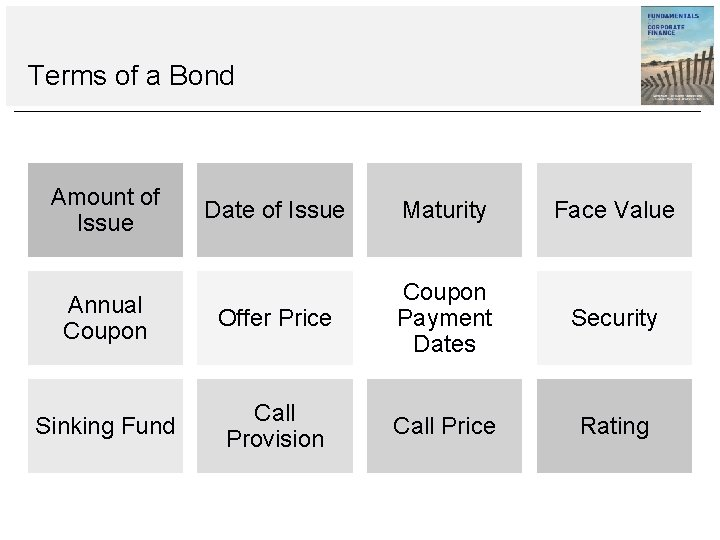Terms of a Bond Amount of Issue Date of Issue Maturity Face Value Annual Coupon Offer Price Coupon Payment Dates Security Sinking Fund Call Provision Call Price RatingBond RatingsDifferent Types of Bond Government Bonds Zero Coupon Bonds Floating Rate Bonds Exotics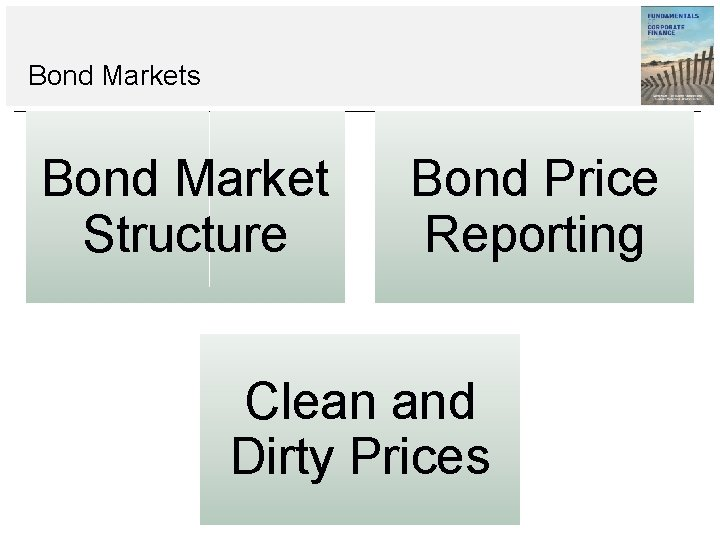Bond Markets Bond Market Structure Bond Price Reporting Clean and Dirty Prices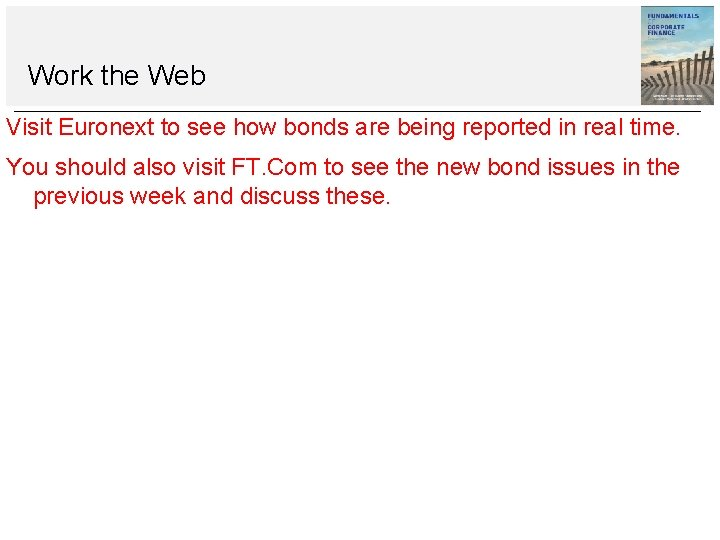Work the Web Visit Euronext to see how bonds are being reported in real time. You should also visit FT. Com to see the new bond issues in the previous week and discuss these.Inflation and Nominal Rates Real Rate Nominal Rate • Interest rate or rate of return that has been adjusted for inflation. • The percentage change in your buying power. • Interest rate or rate of return that has not been adjusted for inflation. • The percentage change in the amount of cash you have.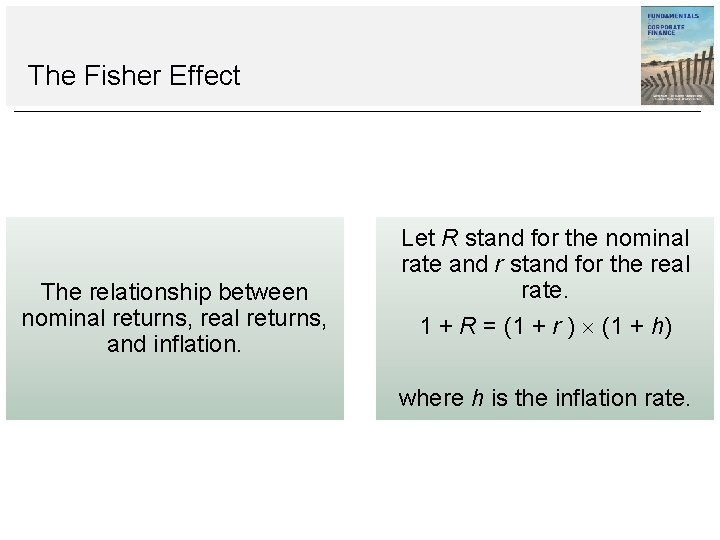The Fisher Effect The relationship between nominal returns, real returns, and inflation. Let R stand for the nominal rate and r stand for the real rate. 1 + R = (1 + r ) (1 + h) where h is the inflation rate.Example 6. 4 The Fisher Effect If investors require a 10 per cent real rate of return, and the inflation rate is 8 per cent, what must be the approximate nominal rate? The exact nominal rate?Example 6. 4 The Fisher Effect From the Fisher effect, we have: Therefore, the nominal rate will actually be closer to 19 per cent.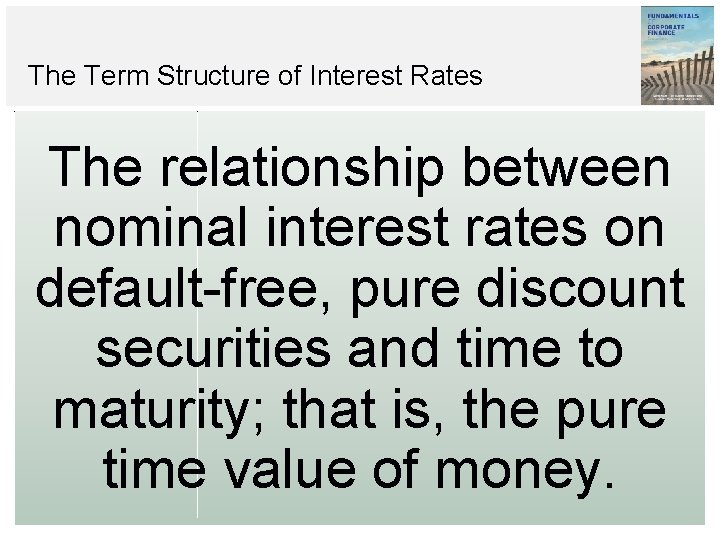The Term Structure of Interest Rates The relationship between nominal interest rates on default-free, pure discount securities and time to maturity; that is, the pure time value of money.Terminology Inflation premium The portion of a nominal interest rate that represents compensation for expected future inflation. Interest rate risk premium The compensation investors demand for bearing interest rate risk.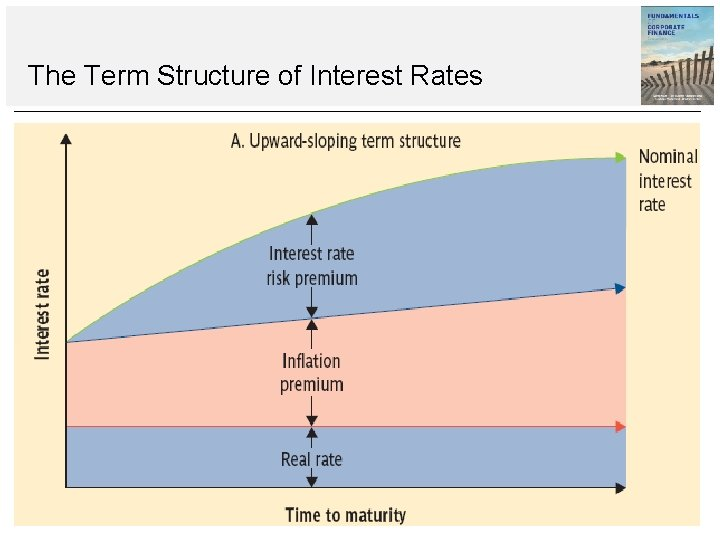The Term Structure of Interest Rates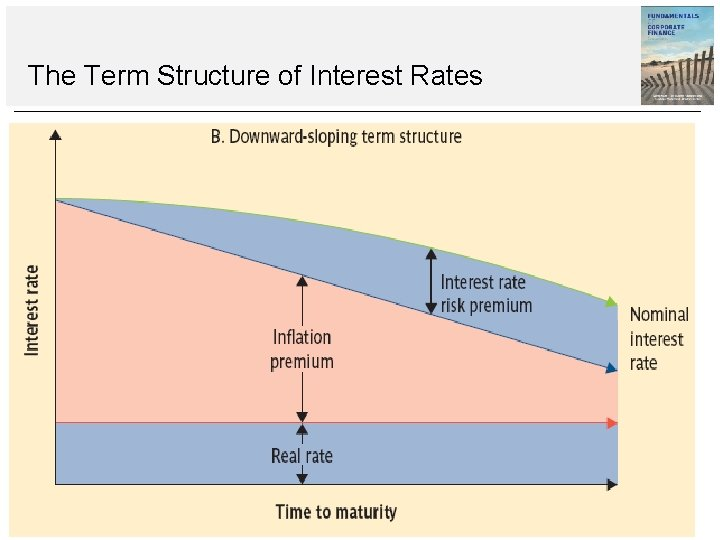The Term Structure of Interest RatesBond Yields and the Yield Curve Treasury yield curve A plot of the yields on Treasury notes and bonds relative to maturity. default risk premium The portion of a nominal interest rate or bond yield that represents compensation for the possibility of default.Work the Web You should visit FT. Com to discuss the most current Yield Curve for your region.Activities for this Lecture Reading • Insert here Assignment • Insert hereThank You